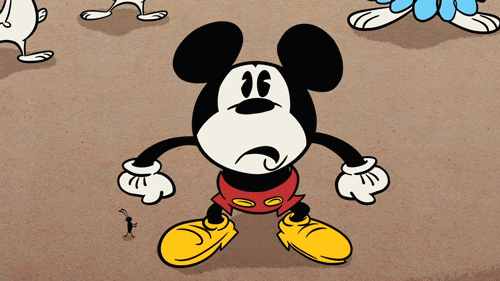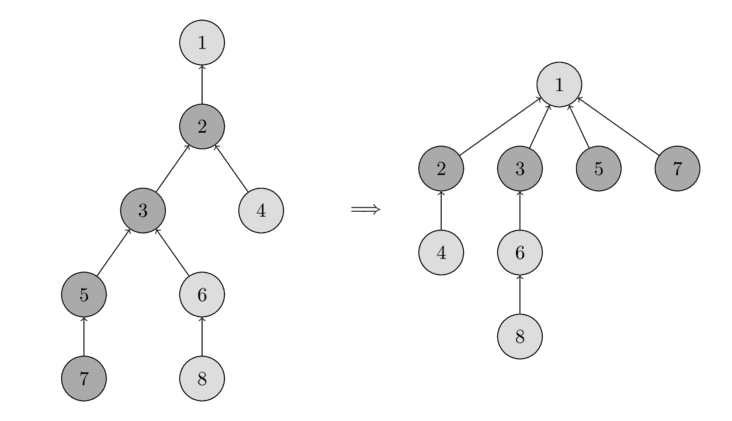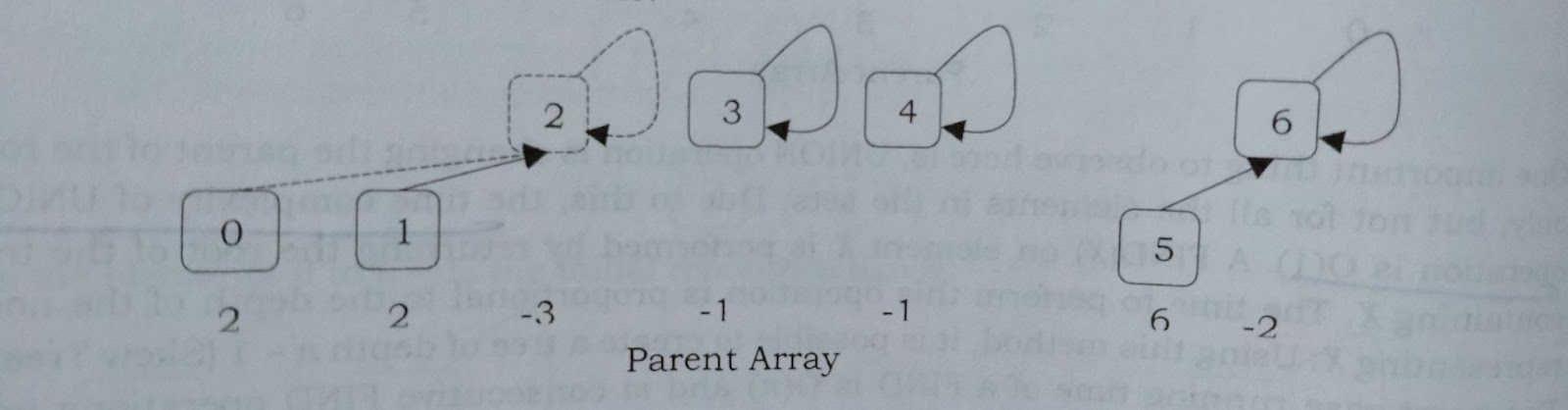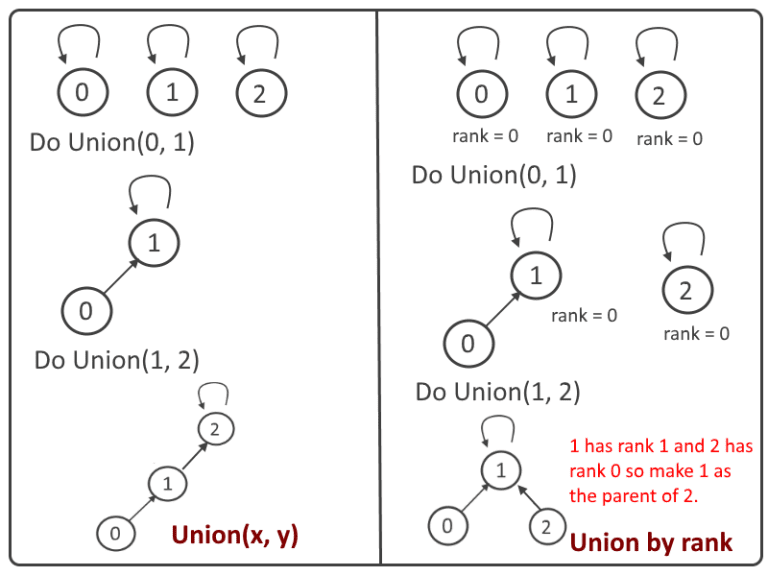# Disjoint Set Unions - Union Find Algorithm

## Introduction

The efficiency of a data structure depends on how efficiently it handles the query of some problem statement. A good choice of data structure can reduce the execution time and that is what comes in handy in real-life problems.
Disjoint Set Union (DSU) is one such data structure. It is also referred to as Union Find because of the functionalities it provides. Today we will discuss Disjoint Set Union or you can say Union Find algorithm from scratch for your better understanding.
Let’s get started with what are disjoint sets?

## Disjoint Sets

To understand disjoint sets, let us break the term into two words - disjoint and sets.

What does disjoint mean? Something which isn’t joined, right?

Now, what is the first thing that comes to our mind when we hear the word sets?

All of us are probably thinking of something like this: S = {1, 2, 3, 4, 5}.

So to understand disjoint sets, let us consider a game of polo. In a game of polo, there are four members on each team. Each member is assigned a jersey number based on the position in which they are playing.

Let us represent this using a graph, where each player is a vertice. For simplicity, I have considered the players 1-4 on the orange team to be numbered from 5-8.

The players in each team can be represented by sets too.

P = {1, 2, 3, 4} and O = {5, 6, 7, 8}

Now, a player of team Purple will not be a player of team orange, so  P ∩ O = Φ. In other words, the two sets are disjoint.

Thus,

Sets that have no elements in common are known as disjoint sets.

Two operations that can be performed on these sets are- find and union. Let us understand what they are one by one.

## Find

Suppose player 1 in the orange team is our friend, and we want to find them on the field during the match. So we will skim through all the players on the field to find them.

Similarly, for two disjoint sets, the find operation is used to find a particular element.

## Union

Let us assume that the Purple and Orange teams are the best polo teams from our state. Now for a national competition, we need to send eight players to represent the state. Since the Purple and Orange teams are the best, we will combine them and send them as a team. This describes the Union operation.

Graphically, we will add an edge between the two disconnected graphs to get the union as shown below:

Mathematically, first, we will take two vertices and see whether they are from different sets.

For example, let us take 4 and 8. 4 belongs to the set P, and 8 belongs to set O. So, we can add an edge between them to find the Union set.

Now the question comes how we will implement the above operations?Source: giphy

Disjoint Set is built as follows:

We will store the sets as trees, with each tree corresponding to one set. And the tree's root will be the set's leader.

Such trees are depicted in the image below.

• Each element begins as a single set which means each vertex is its own tree.
• First, we check if 1 and 2 belong to the same set or not using FIND.
• find_set(x): Determine which subset a particular element belongs to. This can be used to see if two elements are in the same subset.
• Since they are not in the same set. Then we combine the sets containing elements 1 and 2 by using UNION.
• union(x,y): The two specified sets are combined (the set in which the element x is located, and the set in which the element y is located)
• Similarly, we combine the sets containing elements 3 and 4. Finally, we combine the sets containing elements 1 and 3.

From the above figure, we can depict we need something to keep track of the set’s leader. Thus we maintain an array parent that stores a reference to its tree's immediate ancestor.

As a result, this data structure's basic interface will be as follows:

make_set(v)- To make a new set, we simply construct a tree with its root in vertex v, indicating that it is its own ancestor.

find_set(v)- we simply climb the ancestors of the vertex v until we reach the root, i.e. a vertex where the reference to the ancestor leads to itself. This operation can be easily implemented in a recursive manner.

union(a, b)- To combine two sets, we must first identify the representative of the set in which an is located and the representative of the set in which b is located. If the representatives are the same, there is nothing we can do because the sets have already been merged. Otherwise, we can simply specify that one of the representatives is the parent of the other representative, resulting in the two trees being combined.

Here is the implementation of the naive approach:

``````void make_set(int v) {
parent[v] = v;
}

int find_set(int v) {
if (v == parent[v])
return v;
return find_set(parent[v]);
}

void union_sets(int a, int b) {
a = find_set(a);
b = find_set(b);
if (a != b)
parent[b] = a;
}``````

However, this implementation is inefficient.

• One important thing to observe here is, UNION operation is changing the root’s parent only, but not for all the elements in the sets. Due to this, the time complexity of UNION operation is O(1).
• A find_set(x) on element x is performed by returning the root of the tree containing x. The time to perform this operation is proportional to the depth of the node representing x.
• Using this method, it is possible to create a tree of depth n - 1 (Skew Trees). The worst-case running time of a FIND is O(n) and m consecutive FIND operations take O(mn) time in the worst case

There are two ways to improve it:

1. Path Compression
2. Union by Rank

## Path Compression

Path compression is a method of flattening the tree structure when find_set(x) is used on it.

This optimization is designed for speeding up the find_set(x) operation.

If we call find_set(x) for some vertex x, we actually find the representative p for all vertices that we visit on the path between x and the actual representative p.

Here the trick is to make the paths for all those nodes shorter, by setting the parent of each visited vertex directly to p.

Therefore, the resulting tree is much flatter, which speeds up future operations on these elements as well as those that reference them.

Consider the below diagram for better understanding:The left side shows a tree whereas the right side shows a compressed tree for visited nodes 2,3,5,7.

### Implementation

The new implementation of find_set(x) is as follows:

``````int find_set(int v) {
if (v == parent[v])
return v;
return parent[v] = find_set(parent[v]);
}``````

### Complexity Analysis

Time Complexity: O(log N), where n is the number of nodes.

Space Complexity: O(n), where n is the number of nodes.

Let’s move on to other optimization i.e. union by rank without further ado.

## Union by Rank

As we already know when we call find_set(x) and find_set(y) is called it finds the roots that belong to the trees x and y. If the roots of the trees are distinct, the trees are merged by connecting the roots of one to the roots of the other. If this is done carelessly, such as by always making x a child of y, the trees' height can grow as O(n).

We can improve it by employing union by rank.

There are numerous ways that can be taken into account. Here we will discuss the two most popular and most used:

1. Union by rank based on size
2. Union by rank based on depth

The underlying principle of the optimization is the same in both approaches: we attach the tree with the lower rank to the one with the higher rank.

Union by rank based on size

Earlier, we stored i (in the parent array) for the root element and the parent of i for other elements. However, in this approach, we store the reverse of the tree size (that is, if the tree size is 3, we store –3 in the parent array for the root element).

Assume that the size of one element set is 1 and store – 1. Other than this there is no change.### Implementation of Union by rank based on size

``````void make_set(int v) {
parent[v] = v;
size[v] = -1;
}

void union_sets(int a, int b) {
a = find_set(a);
b = find_set(b);
if (a != b) {
if (abs(size[a]) < abs(size[b]))
swap(a, b);
parent[b] = a;
size[a] += size[b];
}
}``````

Union by rank based on depths

In contrast with UNION by size, in this method, we store the depths instead of height because path compression will change the trees’ heights over time.

Each element is associated with a rank. Initially, a set has one element and a rank of zero.### Implementation of Union by rank based on Depth of the trees

``````void make_set(int v) {
parent[v] = v;
rank[v] = 0;
}

void union_sets(int a, int b) {
a = find_set(a);
b = find_set(b);
if (a != b) {
if (rank[a] < rank[b])
swap(a, b);
parent[b] = a;
if (rank[a] == rank[b])
rank[a]++;
}
}``````

Note: For the find_set(x) operation there is no change in the implementation.

### Complexity Analysis

Both optimizations are equivalent in terms of time and space complexity.

Time Complexity: O(log N), where n is the number of nodes.

Space Complexity: O(n), where n is the number of nodes.

So in practice, you can use any of them.

## Code

Now that we know the working of the union-find algorithm, we can write the code for it. I’m sure you can write the code yourself, but here’s the solution of all the operations in one place just in case.

``````// C++ implementation of disjoint set
#include <iostream>
using namespace std;
class DisjointSet {
int *rank, *parent, n;

public:
// Constructor to create and
// initialize sets of n items
DisjointSet(int n)
{
rank = new int[n];
parent = new int[n];
this->n = n;
make_set();
}

// Creates n single item sets
void make_set()
{
for (int i = 0; i < n; i++) {
parent[i] = i;
}
}

// Finds set of given item x
int find_set(int x)
{
// Finds the representative of the set
// that x is an element of
if (parent[x] != x) {

// if x is not the parent of itself
// Then x is not the representative of
// his set,
parent[x] = find_set(parent[x]);

// so we recursively call Find on its parent
// and move i's node directly under the
// representative of this set
}

return parent[x];
}

// Do union of two sets represented
// by x and y.
void Union(int x, int y)
{
// Find current sets of x and y
int xset = find_set(x);
int yset = find_set(y);

// If they are already in same set
if (xset == yset)
return;

// Put smaller ranked item under
// bigger ranked item if ranks are
// different
if (rank[xset] < rank[yset]) {
parent[xset] = yset;
}
else if (rank[xset] > rank[yset]) {
parent[yset] = xset;
}

// If ranks are same, then increment
// rank.
else {
parent[yset] = xset;
rank[xset] = rank[xset] + 1;
}
}
};

int main()
{
DisjointSet obj(5);
obj.Union(0, 2);
obj.Union(4, 2);
obj.Union(3, 1);
if (obj.find_set(4) == obj.find_set(0))
cout << "Yes\n";
else
cout << "No\n";
if (obj.find_set(1) == obj.find_set(0))
cout << "Yes\n";
else
cout << "No\n";

return 0;
}``````

## Applications using Disjoint sets

1. Union-Find algorithm is used to determine the number of connected components in a graph. We can determine whether 2 nodes are in the same connected component or not in the graph. We learned that we can reduce its complexity to a very optimum level, so in case of very large and dense graph, we can use this data structure.
2. Represents network connectivity.
3. Image Processing.
4. In-game algorithms.
5. Kruskal’s minimum spanning tree- Union Find Data Structure is used as a subroutine to find the cycles in the graph, which helps in finding the minimum spanning tree.
6. Find cycle in undirected graphs.

Watch this video tutorial to understand the concept more deeply and make your concept stronger.

1. Where is the union-find algorithm most commonly used?
The union-find algorithm is most commonly used to detect cycles in a graph in Kruskal’s algorithm.
2. Which algorithm uses the union-find algorithm in disjoint sets?
Kruskal’s algorithm uses the union-find algorithm in disjoint sets.
3. Can the union-find algorithm be used in disjoint sets only?
Yes, the union-find algorithm is used in disjoint sets only.
4. What is the time complexity of the union-find algorithm?
The time complexity of the union-find algorithm is less than O(log n) and is often constant.

## Key takeawaysNow that we know the union-find algorithm, understanding Kruskal’s algorithm will be easier!

So what are we waiting for?

Let’s learn about Kruskal’s algorithm next!